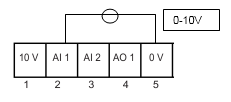# 以下信息适用范围：SINAMICS V20P0756 = 0 ；模拟量输入类型，电压类型
P0757 = 0 ；标定X1值 = 0V
P0758 = 0.0 ；标定Y1值 = 0%
P0759 = 10 ；标定X2值 = 10V
P0760 = 100.0 ；标定Y2值 = 100%
P0761 = 0 ，模拟量输入死区

P1000 = 2 ，使用模拟量输入1调节变频器输出频率